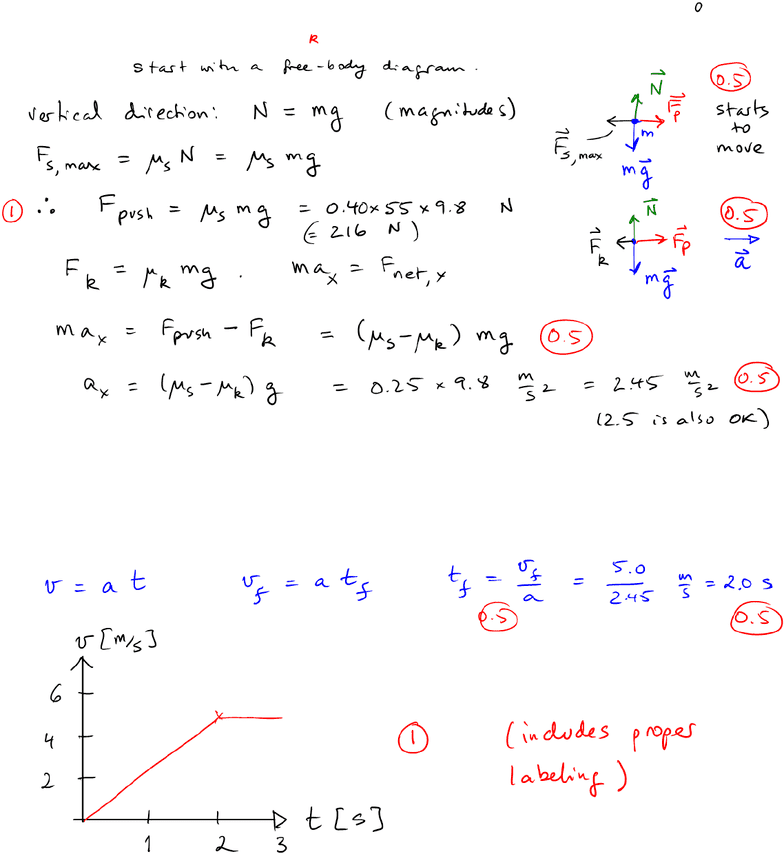# PHYS 1010 Study Guide - Midterm Guide: Terminal Velocity, Antiderivative, Friction

80 views4 pagesLAST NAME: STUDENT NR:
PHYS 1010 6.0: CLASS TEST 1
Time: 50 minutes; Calculators & formulae provided at the end = only aid; Total = 20 points.
1 a) The coeﬃcient of static friction between a crate and the (level) ﬂoor is given as µs= 0.4,
while the kinetic friction coeﬃcient is µs= 0.15. The mass of the crate is m= 55kg. You apply
a force that is just enough to make the refrigerator move. What is the acceleration? Explain
1 b) The maximum speed at which you will push this crate is vf= 5.0m/s. At which time
will you reach this speed? Provide a velocity vs time graph from the start, and past the point
of reaching vf
1
Unlock document

This preview shows page 1 of the document.
Unlock all 4 pages and 3 million more documents.

## Document Summary

Time: 50 minutes; calculators & formulae provided at the end = only aid; total = 20 points. 1 a) the coe cient of static friction between a crate and the (level) oor is given as s = 0. 4, while the kinetic friction coe cient is s = 0. 15. The mass of the crate is m = 55kg. You apply a force that is just enough to make the refrigerator move. 1 b) the maximum speed at which you will push this crate is vf = 5. 0m/s. Provide a velocity vs time graph from the start, and past the point of reaching vf. 2 a)  a girl (mass m) is jumping from a ladder. Indicate the forces with arrows of appropriate respective length in the fb diagrams, and also the acceleration of the centre of gravity (beside the diagrams). 7. 8 103, glass = 2. 5 103, lead = 1. 1 104.